# GRE Math Review Quiz: Plugging In

I’ve compiled 7 practice problems (some old, some more recent) from math posts on our blog that are great practice for using the “plugging in” strategy.  If you’re already familiar with the approach, test yourself with these problems to review the strategy– it’s a method you’ll definitely be able to use for several different question types in GRE math.

1. A transcontinental jet travels at a rate of  x–100 mph with a headwind and x+100 mph with a tailwind between Wavetown and Urbanio, two cities 3,200 miles apart. If it takes the jet 2 hr 40 minutes longer to complete the trip with a headwind, then what is the jet’s rate flying with a tailwind?

1. 500
2. 540
3. 600
4. 720
5. Cannot be determined by the information given.

Answer and explanation: GRE Math Strategies: When to Plug In

2. Xyla can paint a fence in 6 hrs. Working alone, Yarba can paint the same fence in x hours. Working together they can complete the task in 2.1 hrs.

 Column A Column B x 3

1. The quantity in Column A is greater
2. The quantity in Column B is greater
3. The two quantities are equal
4. The relationship cannot be determined from the information given

Answer and explanation: Does Plugging In work on GRE Quantitative Comparison?

3. A concession stand sells either hotdogs for \$1.75 each or hamburgers for \$4 each. If Charlie buys a total of 9 items from the concession stand for a total of 27 dollars, then how many hot dogs did he buy?

1. 3
2. 4
3. 6
4. 7
5. 8

Answer and explanation: Part I: The Power of Plugggin In– GRE Math Techniques

4. If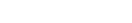, what is the value of x?

1.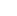2.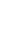3.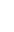4.5.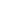Answer and explanation: GRE Exponents: Basics & Exponent Practice Question Set

5. Which of the following must be greater than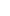, where -1 < x < 0?  Choose ALL that apply.

1.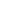2.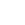3.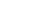4.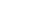5.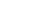6. ^m^ is equal to the digits in positive integer m in reverse order, discounting the zeroes (e.g. ^41^ = 14 but ^3500^ = 53). Which of the following must be true? Select all that apply.

1. ^m^ < ^m+1^
2. m = ^(^m^)^
3. ^1000m^ = ^m^
4. (^m^)(^m^) > ^m^
Answer and Explanation: New GRE Math Strategies

7. Charlie takes 2.5 hours to fly from Los Angeles to Mexico City, a distance of 1200 miles. What is the average speed of his plane in miles per hour?
1. 200 mph
2. 240 mph
3. 410 mph
4. 480 mph
5. 533 mph

Answer and explanation: Math Basics: Distance, Rate, and Time

Let us know if you have any questions, good luck!

## Author

•Margarette works on marketing strategy for Magoosh and makes a lot of fun spreadsheets. She graduated from UC Berkeley with a double major in English Literature and Linguistics. Working at Magoosh combines many of her favorite things: using email keyboard shortcuts, finding patterns, and thinking about the future of the Internet. She also enjoys dystopian fiction, Chipotle, and learning about learning.